# Common Core: 7th Grade Math : Solve Word Problems Leading to Inequalities: CCSS.Math.Content.7.EE.B.4b

## Example Questions

← Previous 1 3 4 5

### Example Question #71 : Expressions & Equations

Write as an algebraic inequality:

Twenty subtracted from the product of seven and a number exceeds one hundred.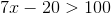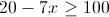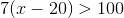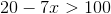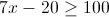Explanation:

"The product of seven and a number " is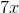. "Twenty subtracted from the product of seven and a number" is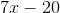. "Exceeds one hundred" means that this is greater than one hundred, so the correct inequality is### Example Question #1 : Writing Inequalities

Write as an algebraic inequality:

Twice the sum of a number and sixteen is no less than sixty.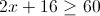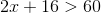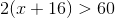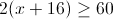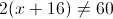Explanation:

"The sum of a number and sixteen" is translates to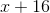; twice that sum is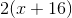. " Is no less than sixty" means that this is greater than or equal to sixty, so the desired inequality is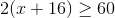.

### Example Question #1 : Writing Inequalities

Write as an algebraic inequality:

Twice the sum of a number and sixteen does not exceed eighty.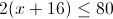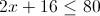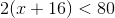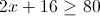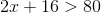Explanation:

"The sum of a number and sixteen" translates to; twice that sum is. "Does not exceed eighty" means that it is less than or equal to eighty, so the desired inequality is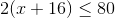### Example Question #1 : Solve Word Problems Leading To Inequalities: Ccss.Math.Content.7.Ee.B.4b

How would you write the equations: "I can spend no more thandollars when I go to the store today."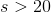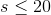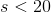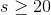Explanation:

The way the sentence is phrased suggests that the person can spend up todollars but not a penny more. This suggests that, the amount spend can bebut not exceed it.

So your answer is:### Example Question #1 : Solve Word Problems Leading To Inequalities: Ccss.Math.Content.7.Ee.B.4b

Given the following problem, write the inequality.

Seven less than two times a number is greater than fourteen.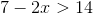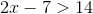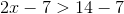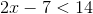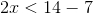Explanation:

### Seven less than two times a number is greater than fourteen.

Let's look at the problem step by step.

If we do not know the value of a number, we give it a variable name.  Let's say x.  So, we see in the problem

Seven less than two times a number is greater than fourteen.

So, we will replace a number with x.

Seven less than two times x is greater than fourteen.

Now, we see that is says "two times" x, so we will write it like

Seven less than 2x is greater than fourteen.

The problem says "seven less" than 2x.  This simply means we are taking 2x and subtracting seven.  So we get

2x - 7 is greater than fourteen

We know the symbol for "is greater than".  We can write

2x - 7 > fourteen

Finally, we write out the number fourteen.

2x - 7 > 14

### Example Question #1 : Solve Word Problems Leading To Inequalities: Ccss.Math.Content.7.Ee.B.4b

Express the following as an inequality:

Bob's amount of apples () is more than twice the amount of Adam's bananas ().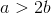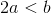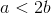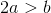Explanation:

To solve, you must convert the statement into an expression. The key work is "is". Whatever is on the left of that in the sentence will be on the left side of the expression. The same goes for the right. Thus,is on the left and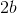is on the right.### Example Question #12 : Writing Inequalities

Write the following as a mathematical inequality:

A number is less than or equal to three times the sum of another number and five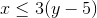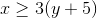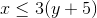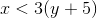Explanation:

Write the following as a mathematical inequality:

A number is less than or equal to three times the sum of another number and five.

Let's begin with

"A number" let's call it x

"...is less than or equal to..."

So far we have: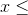Now,

"...three times..."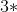"...the sum of another number and five."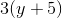So, all together:### Example Question #1 : Solve Word Problems Leading To Inequalities: Ccss.Math.Content.7.Ee.B.4b

Write the inequality:

Two less than twice a number is less than two.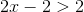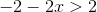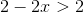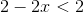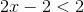Explanation:

Break up the statement by parts.  Let that number be.

Twice a number:Two less than twice a number: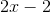Less than two: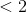Combine the parts.

The answer is:### Example Question #14 : Writing Inequalities

Write the inequality:  Three less than twice a number is more than three times the number.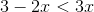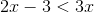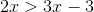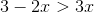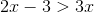Explanation:

Break up the sentence into parts.  Let the number be.

Twice a number:Three less than twice a number: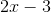Three less than twice a number is more than: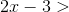Three times the number:Combine the terms to form the inequality.

The answer is:### Example Question #1 : Solve Word Problems Leading To Inequalities: Ccss.Math.Content.7.Ee.B.4b

Write the inequality:  A number less than three is less than three.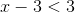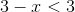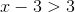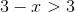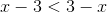Explanation:

Let a number be.  Split up the problem into parts.

A number less than three: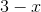Is less than three: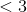Combine all the terms.

The answer is:← Previous 1 3 4 5

### All Common Core: 7th Grade Math Resources